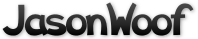Got questions, comments, patches, etc.? Contact Jason Woofenden
 author Jason Woofenden Sat, 2 Nov 2013 18:26:14 +0000 (14:26 -0400) committer Jason Woofenden Sat, 2 Nov 2013 18:26:14 +0000 (14:26 -0400)
 main.coffee patch | blob | history t patch | blob | history

index 1847e56..b44afb6 100644 (file)
@@ -26,6 +26,8 @@ slide_ms = 2000

board_col_heights = [5, 6, 7, 8, 7, 6, 5]

+# code and css will need adjusting if you change HP_MAX
+HP_MAX = 10

##############################################################
##############    fix javascript some more    ################
@@ -231,7 +233,7 @@ init_board = ->
if encoded? and encoded.length > board_tiles
new_letter_queue = (encoded.substr 0, board_tiles).split ''
-               score = parseInt(encoded.substr(board_tiles), 10)
+               score = parseInt(encoded.substr(board_tiles), HP_MAX)

# work out which grid spaces are connected
# (neighbors, above, down)
@@ -311,17 +313,53 @@ save_game = ->

# remove the selected tiles from the board, create new tiles, and slide everything into place
blip_selection = ->
+       difficulty = 7 # higher numbers are easier
+       unsink = difficulty * score_for selected_word() # how much tile restoration we have left to do
selected = []
update_selection_display()
+       neighbors = {}
+       nneighbors = {}
+               tiles[i].new_hp = tiles[i].hp
+               for n in board_neighbors[i]
+                       neighbors[n] = tiles[n]
+                       for nn in board_neighbors[n]
+                               nneighbors[nn] = tiles[nn]
+               delete nneighbors[i]
+               delete neighbors[i]
+       for k, v of neighbors
+               delete nneighbors[k]
+       # convert to arrays so we can sort, etc
+       nneighbors = (v for k, v of nneighbors)
+       neighbors = (v for k, v of neighbors)
+       # TODO randsort neighbors and nneighbors
+       for nei in [neighbors, nneighbors]
+               if unsink > 0
+                       for i in nei
+                               if i.hp is 0 and unsink >= 15
+                                       i.new_hp = 10
+                                       unsink -= 15
+                                       i.text = new_letter()
+                                       i.dom.html i.text
+               if unsink > 0
+                       for i in nei
+                               if i.hp > 0 and unsink > 0
+                                       unsink -= 10 - i.hp
+                                       i.new_hp = 10
for i in tiles
-                       unless i.hp < 1
-                               i.dom.removeClass "hp#{i.hp}"
-                               i.hp -= 1
+               i.new_hp ?= i.hp - 1
+               if i.new_hp < 0
+                       i.new_hp = 0
+               else if i.new_hp > HP_MAX
+                       i.new_hp = HP_MAX
+               if i.new_hp isnt i.hp
+                       i.dom.removeClass "hp#{i.hp}"
+                       i.hp = i.new_hp
+               delete i.new_hp
# which tiles need to be slid down
sliders = (false for i in tiles)
@@ -407,11 +445,13 @@ select_tile = (num) ->
new_tile = (num, x, y) ->
letter = new_letter()

-       html_tile = \$("<div class=\"tile hp10\" style=\"left: #{x}px; top: #{y}px\" unselectable=\"on\">#{letter}</div>")
+       hp = Math.floor(Math.random() * HP_MAX)
+
+       html_tile = \$("<div class=\"tile hp#{hp}\" style=\"left: #{x}px; top: #{y}px\" unselectable=\"on\">#{letter}</div>")
\$board.append(html_tile)

html_tile.data 'tile_number', num
-       tiles[num] = text: letter, dom: html_tile, hp: 10, id: num
+       tiles[num] = text: letter, dom: html_tile, hp: hp, id: num

html_tile.click ->
me = \$(this)
@@ -620,14 +660,10 @@ init_keybinding = ->
when 27
unselect_all()

-\$log = undefined
-init_log = ->
-       \$log = \$('#log')
log = (msg) ->
-       console.log msg if console.log?
+       console.log msg if console?.log?

init_game = ->
-       init_log()
if \$(window).height() >= 440
\$('#centerer').css('margin-top', '25px')
init_keybinding()
diff --git a/t b/t
index c796533..711f21c 100755 (executable)
--- a/t
+++ b/t
@@ -3,5 +3,10 @@
while true
do
inotifywait -q -e close_write -e delete_self `git ls-files` >/dev/null 2>&1 || exit
-       verbose make #&& dwb -x reload
+       if verbose make; then
+               notify-send "hexbog" "built"
+       else
+               notify-send "hexbog" "error building"
+       fi
+       #verbose make && dwb -x reload
done